Question

B4 Which of the following is not part of the South African Reserve Bank’s monetary policy framework?
 inflation targeting.
 maintaining price stability
 influencing interest rate levels
 achieving sustainable economic growth.
 all of the above form part of the South African Reserve Bank’s monetary policy framework.

B5 Which of the following statements regarding the foreign sector is/are correct?
a. Absolute advantage is a prerequisite for international trade.
b. Differences in resource endowments necessitate international trade.
c. Countries can only benefit from trade if the opportunity costs among the trading countries are the same.
 Only a and b
 Only and c
 Only b and c
 Only b
 Only c

B8 A decrease in the demand for USA dollar (\$) in the South African foreign exchange market would result if there is
 a decrease in South African exports to the USA.
 a decrease in the gold price in South Africa.
 a decrease in South African tourists to the USA.
 a decrease in USA tourists to South Africa.

B9 Other things equal, recessions in the economies of South Africa’s trading partners will
 have no perceptible impact on the South African economy.
 cause inflation in the South African economy.
 depress real output and employment in the South African economy.
 stimulate real output and employment in the South African economy.
 None of the statements are correct.

B10 If the SARB buys dollars in the South African foreign exchange market, the South African rand will
 not be affected
 appreciate
 depreciate

B11 When the rand appreciates against the dollar, it
a. appreciates against other currencies as well.
b. stimulates the exports and dampens the imports.
c. improves the balance of payments.
 None of the statements are correct.
 Only a and c
 Only b and c
 Only b
 Only c

B12 In the simple Keynesian model,
 production is always equal to aggregate expenditure
 aggregate expenditure is less than production
 aggregate demand determines production
 aggregate supply determines production

B13 Which one of the following statements regarding Say`s law is correct?
 overproduction is not possible
 demand determines supply
 aggregate spending determines production
 consumption is equal to savings

B14 Which one of the following statements regarding the assumptions of the Keynesian model is correct?
 the simple Keynesian model can be used to study inflation
 the simple Keynesian model can be used to explain the workings of the labour market.
 the simple Keynesian model can be used to study monetary policy
 in the simple Keynesian model prices, wages, money supply and the interest rate are given meaning that their value is determined outside the model.

B20 To get the equilibrium level of income in the simple Keynesian model
 we multiply the autonomous aggregate spending by the multiplier
 we add all the autonomous aggregate spending component and subtract the multiplier
 we divide the multiplier by aggregate demand
 we multiply the interest rate by the multiplier

B21 An increase in the tax rate in the Keynesian model will
 shift the aggregate spending curve upwards in a parallel way
 shift the consumption curve upwards in a parallel way
 not affect the aggregate spending curve
 swivel the aggregate demand curve downwards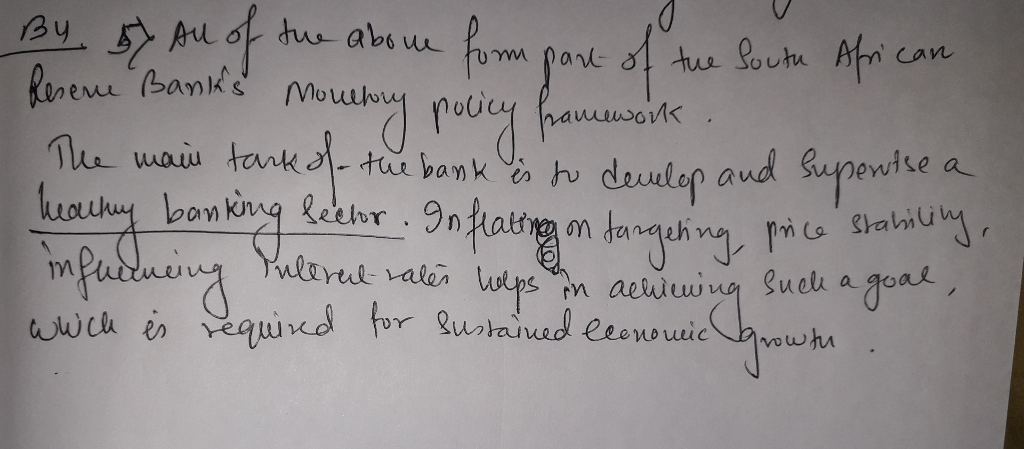#### Earn Coins

Coins can be redeemed for fabulous gifts.

Similar Homework Help Questions
• ### Section A B3 Which of the following statements regarding taxation is incorrect?  As interest rates...

Section A B3 Which of the following statements regarding taxation is incorrect?  As interest rates increase, bond prices decrease.  As interest rates decrease, bond prices increase.  There is a positive relationship between the interest rate and bond prices.  If interest rates are high, the quantity of money demanded will tend to be low.  If interest rates are low, the quantity of money demanded will tend to be high. B4 Which of the following is not...

• ### Note: There is only one correct option. 1. To get the equilibrium level of income in...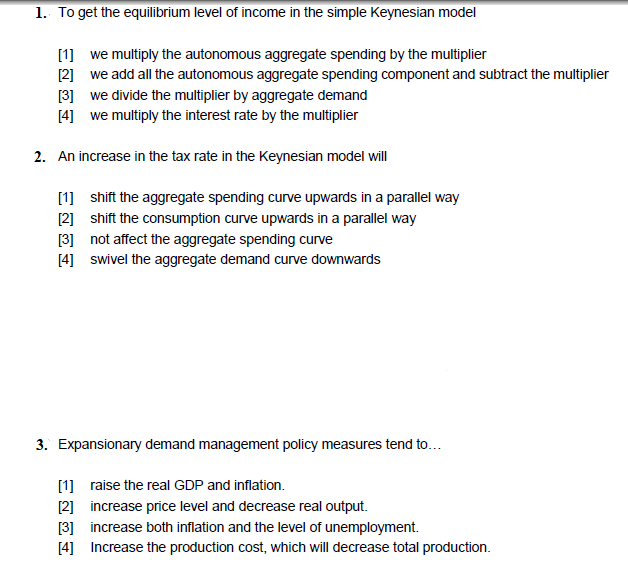Note: There is only one correct option. 1. To get the equilibrium level of income in the simple Keynesian model  we multiply the autonomous aggregate spending by the multiplier 12 we add all the autonomous aggregate spending component and subtract the multiplier  we divide the multiplier by aggregate demand  we multiply the interest rate by the multiplier 2. An increase in the tax rate in the Keynesian model will 1 shift the aggregate spending curve upwards in...

• ### 1) of the Central Bank of Kuwait puts in place an expansionary monetary policy, its decision...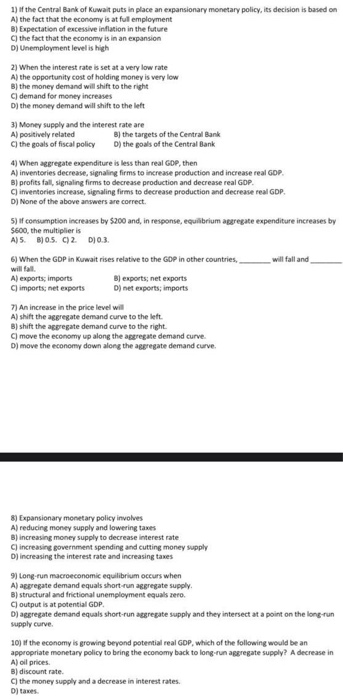1) of the Central Bank of Kuwait puts in place an expansionary monetary policy, its decision is based on A) the fact that the economy is at ful employment B) Expectation of excessive inflation in the future C) the fact that the economy is in an expansion D) Unemployment level is high 2) When the interest rate is set at a very low rate A) the opportunity cost of holding money is very low B) the money demand will shift...

• ### Note: Only select the correct option 1. Which of the following statements regarding the foreign sector...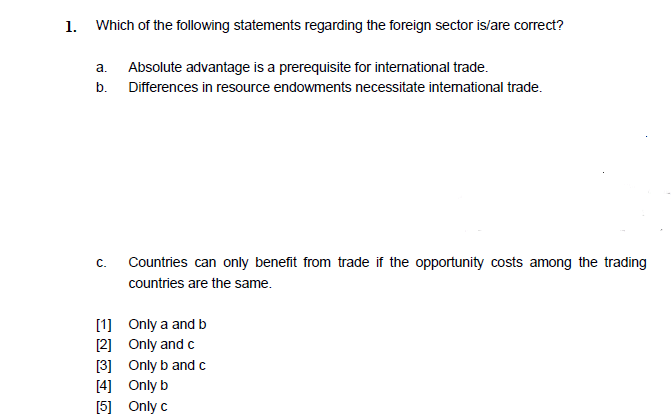Note: Only select the correct option 1. Which of the following statements regarding the foreign sector is/are correct? a. Absolute advantage is a prerequisite for international trade. b. Differences in resource endowments necessitate intemational trade. Countries can only benefit from trade if the opportunity costs among the trading countries are the same. c.  Only a and b 2 Only and c 3 Only b and c 4 Only b 2. Which one of the following statements regarding the assumptions...

• ### Which of the following is a monetary policy intended to rein in inflation? a. Reduce interest...

Which of the following is a monetary policy intended to rein in inflation? a. Reduce interest rates to increase investment spending b. Increase the money supply to shift the aggregate demand curve rightward c. Reduce the interest paid on banks' reserves d. Decrease the money supply to shift the aggregate demand curve leftward

• ### 1. A movement to the right (upward) along the intermediate range of the Modern Keynesian aggregate...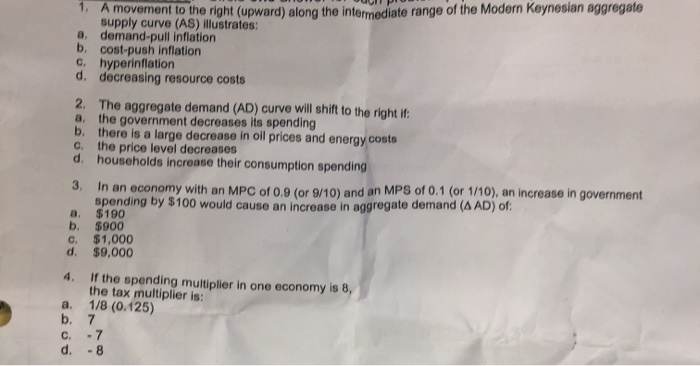1. A movement to the right (upward) along the intermediate range of the Modern Keynesian aggregate supply curve (AS) illustrates: a. demand-pull inflation b. cost-push inflation C, hyperinflation d. decreasing resource costs 2. The aggregate demand (AD) curve will shift to the right if a. the government decreases its spending b. there is a large decrease in oil prices and energy costs c. the price level decreases d. households increase their consumption spending In an economy with an MPC of...

• ### what are the primary objective of South African reserve bank formulate and implement monetary policies 2....

what are the primary objective of South African reserve bank formulate and implement monetary policies 2. maintain financial stability.3 all of above 4 none of above

• ### f contractionary monetary policy is used, then which of the following would be most likely to...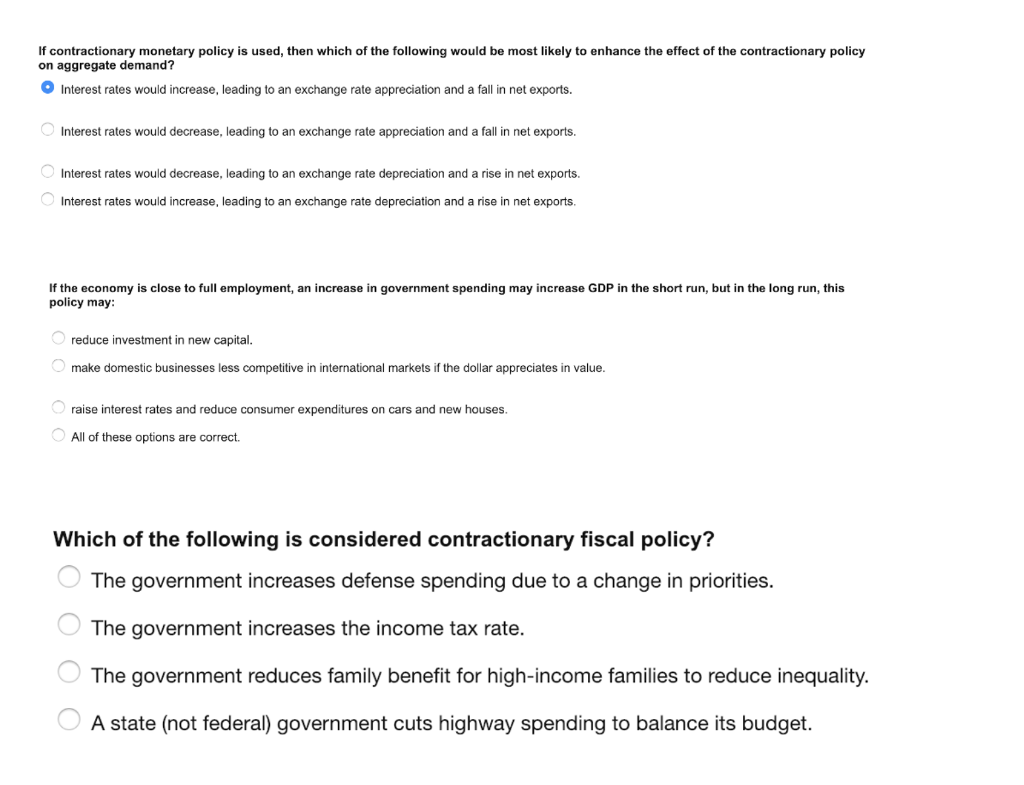f contractionary monetary policy is used, then which of the following would be most likely to enhance the effect of the contractionary policy on aggregate demand? Interest rates would increase, leading to an exchange rate appreciation and a fall in net exports. Interest rates would decrease, leading to an exchange rate appreciation and a fall in net exports. Interest rates would decrease, leading to an exchange rate depreciation and a rise in net exports. Interest rates would increase, leading to...

• ### (1) Other things being equal, which of the following will increase aggregate expenditures? Group of answer...

(1) Other things being equal, which of the following will increase aggregate expenditures? Group of answer choices An increase in domestic prices relative to foreign prices A decrease in the interest rate A decrease in real wealth An increase in income taxes A decrease in government purchases of goods and services (2) If the current unemployment rate is 5 percent and the natural unemployment rate is 6 percent, then the economy is Group of answer choices producing a level of...

• ### Which of the following are ways that the Federal Reserve influences the U.S. economy through its monetary policies?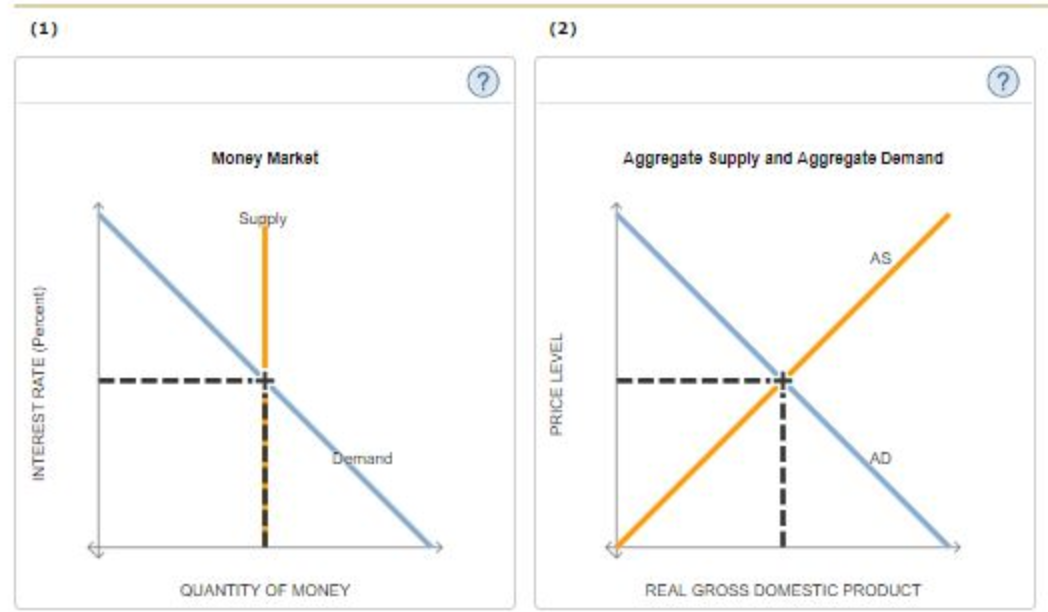3. How the Fed influences the money supply Which of the following are ways that the Federal Reserve influences the U.S. economy through its monetary policies? Check all that apply. O Using open-market operations to sell securities, the Fed can increase the money supply, thereby increasing interest rates and subsequently reducing the rate of inflation. O Using open-market operations to buy securities, the Fed can increase the money supply, thereby increasing interest rates, which would cause security prices to decrease. Using open-market operations to sell...